# Stop Thinking, Just Do!

Sung-Soo Kim's Blog

# Predicting Car Prices Part 1: Linear Regression## 1 Introduction

Let’s walk through an example of predictive analytics using a data set that most people can relate to:prices of cars. In this case, we have a data set with historical Toyota Corolla prices along with related car attributes.

Let’s load in the Toyota Corolla file and check out the first 5 lines to see what the data set looks like:

##   Price Age    KM FuelType HP MetColor Automatic   CC Doors Weight
## 1 13500  23 46986   Diesel 90        1         0 2000     3   1165
## 2 13750  23 72937   Diesel 90        1         0 2000     3   1165
## 3 13950  24 41711   Diesel 90        1         0 2000     3   1165
## 4 14950  26 48000   Diesel 90        0         0 2000     3   1165
## 5 13750  30 38500   Diesel 90        0         0 2000     3   1170


We find the following variables:

• Price
• Age
• KM(kilometers driven)
• Fuel Type
• HP(horsepower)
• Automatic or Manual
• Number of Doors
• Weight(in pounds)

are the data collected in this file for Toyota Corollas. You can download this dataset from my github account here: https://github.com/datailluminations/PredictingToyotaPricesBlog

In predictive models, there is a response variable(also called dependent variable), which is the variable that we are interested in predicting.

The independent variables(the predictors also called features in the machine learning community) are one or more numeric variables we are using to predict the response variable. Given we are using a linear regression model, we are assuming the relationship between the independent and dependent variables follow a straight line. In future posts, we will gradually increase the complexities of our models to see if it improves predictive powers.

But before we start our modeling exercise, it’s good to take a visual look at what we are trying to predict to see what it looks like. Since we are trying to predict Toyota Corolla prices with historical data, let’s do a simple histogram plot to see the distribution of Corolla prices: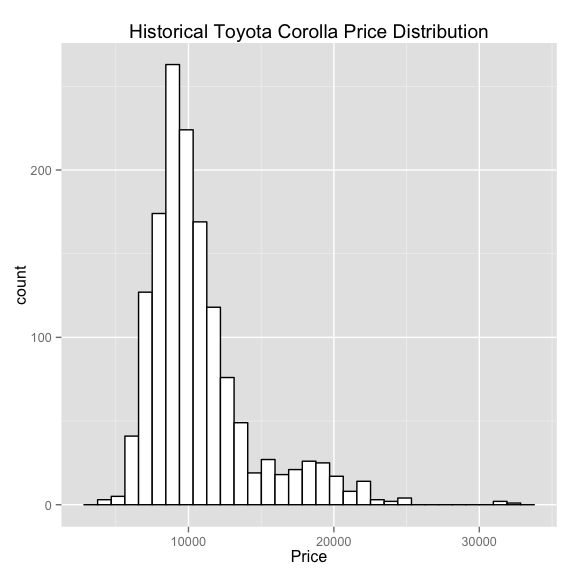We see that most used Corollas are around \$10K and there are some at the tail end that over \$25K. These might be newer cars with a lot of options. And there are fewer of them anyhow.

## 2 Data Transformation:

One of the main steps in the predictive analytics is data transformation. Data is never in the way you want them. One might have to do some kinds of transformations to get it to the way we need them to be either because the data is dirty, not of the type we want, out of bounds, and a host of other reasons.

In this case, we need to convert the categorical variables to numeric variables to feed into our linear regression model, because linear regression models only take numeric variables.

The categorical variable we want to do the transformation on is Fuel Types. We that there are 3 Fuel Types: 1) CNG 2) Diesel 3) Petrol

summary(corolla\$FuelType)
##    CNG Diesel Petrol
##     17    155   1264


So, we can convert the categorical variable Fuel Type to two numeric variables: FuelType1 and FuelType2. We assign CNG to a new variable FuelType1 in which a 1 represents it’s a CNG vehicle and 0 it’s not. Likewise, we assign Diesel to a new variable FuelType2 in which a 1 represents it’s a Diesel vehicle and 0 it’s not.

So, what do we do with PETROL vehicles? This is represented by the case when BOTH FuelType1 and FuelType2 are zero.

##   Price Age    KM HP MetColor Automatic   CC Doors Weight FuelType1
## 1 13500  23 46986 90        1         0 2000     3   1165         0
## 2 13750  23 72937 90        1         0 2000     3   1165         0
## 3 13950  24 41711 90        1         0 2000     3   1165         0
##   FuelType2
## 1         1
## 2         1
## 3         1


## 3 Exploratory Data Analysis (EDA):

The next step in predictive analytics is to explore our underlying. Let’s do a few plots of our explantory variables to see how they look against Price.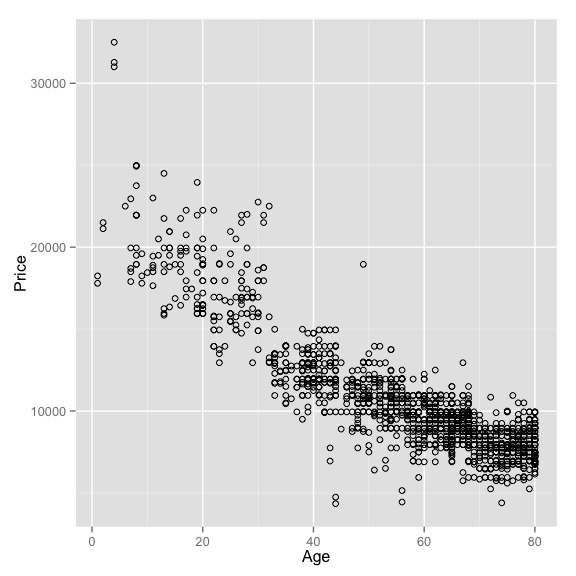This plot is telling and fits out intuition. The newer the car the more expensive it is.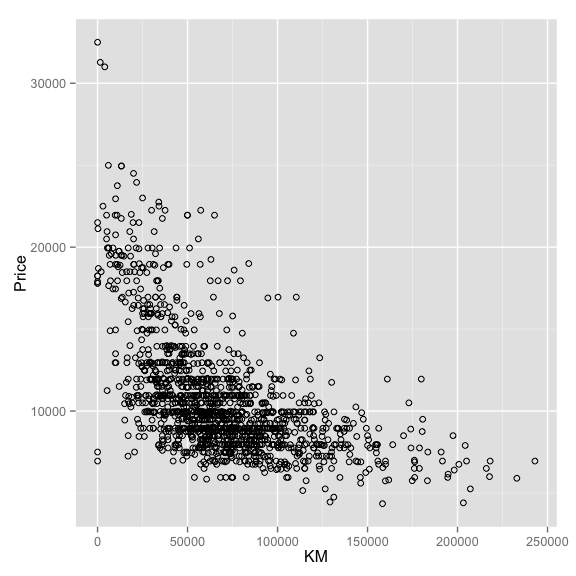The more miles a car has the cheaper it is.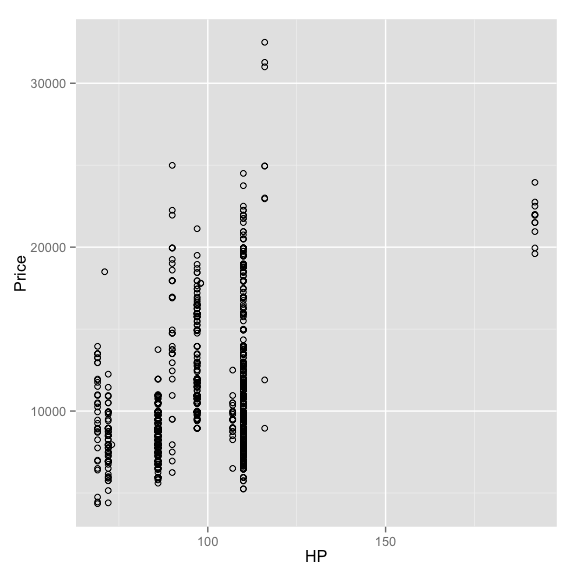This one is not as direct as the other. Yes, the more horsepower the more expensive. But not always the case. Let’s see how this variable will behave in our model.

{r, echo=FALSE}, fig.width=6, fig.height=6
ggplot(auto, aes(x=MetColor, y=Price,color=factor(MetColor))) + geom_point(shape=1)


The fact that a color has a Metallic Color or not doesn’t seem to be that useful. But let’s see what the model says.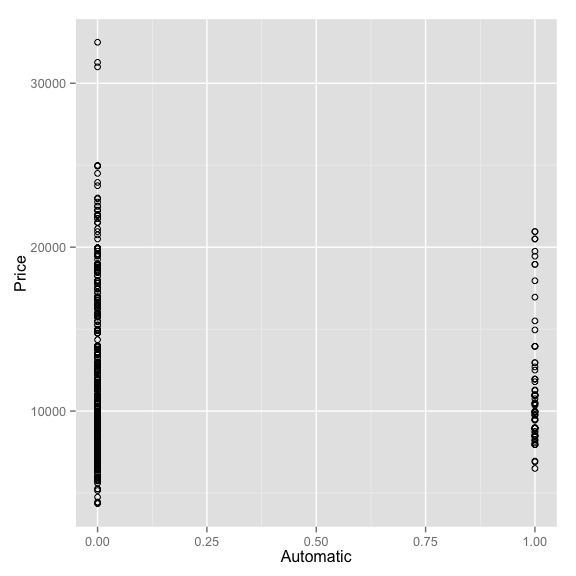What does this tell us about automatic vs manual cars? Not much of an influence to Prices.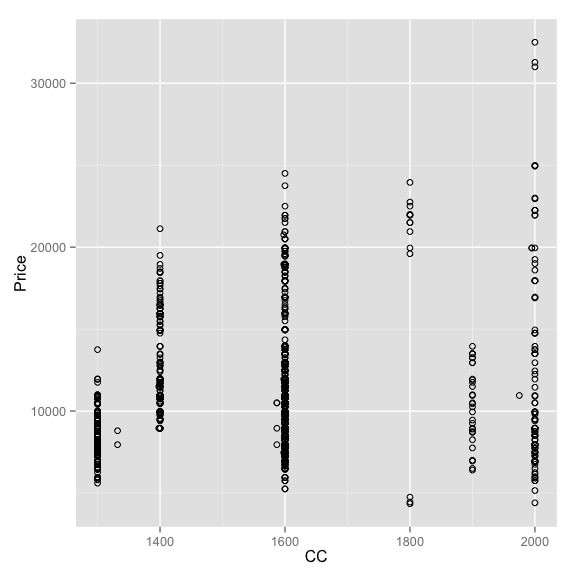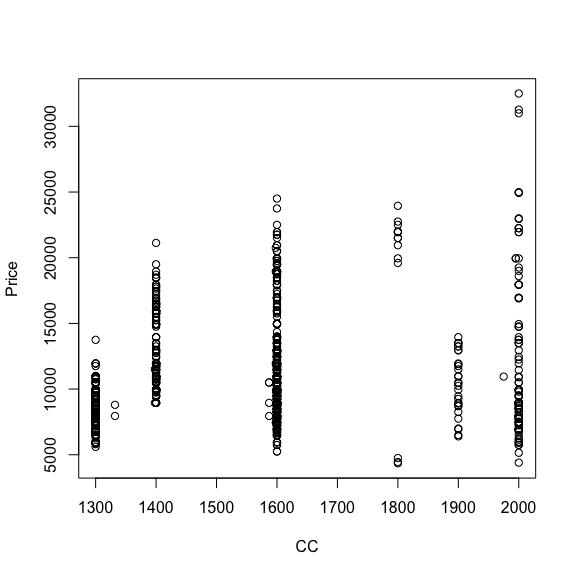The number of cyclinders(CC) plots against Price seems to show the more cyclinder the more expensive though not always the case.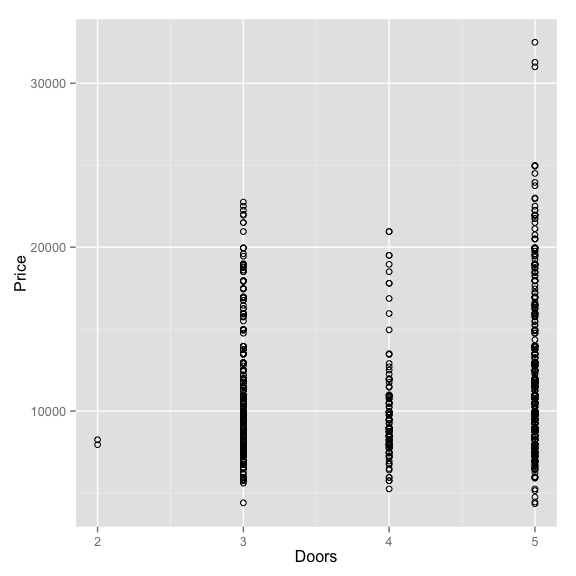What does this tell us about the number of doors as it relates to price of cars? Not much.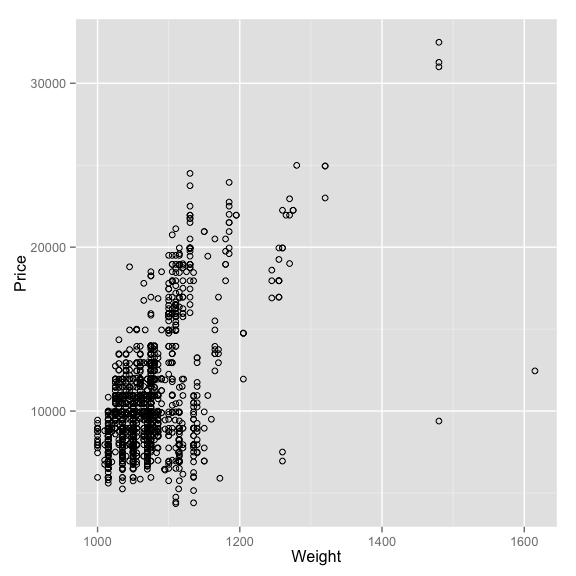This shows the heavier(i.e. bigger) cars cost more though there are some outliers that doesn’t fit nicely.

## 4 Model Building: Linear Regression

Now that we have explored our variables, let’s a simple linear regression of Price against all the data we’ve collected.

##
## Call:
## lm(formula = Price ~ ., data = auto)
##
## Residuals:
##      Min       1Q   Median       3Q      Max
## -10642.3   -737.7      3.1    731.3   6451.5
##
## Coefficients:
##               Estimate Std. Error t value Pr(>|t|)
## (Intercept) -2.681e+03  1.219e+03  -2.199 0.028036 *
## Age         -1.220e+02  2.602e+00 -46.889  < 2e-16 ***
## KM          -1.621e-02  1.313e-03 -12.347  < 2e-16 ***
## HP           6.081e+01  5.756e+00  10.565  < 2e-16 ***
## MetColor     5.716e+01  7.494e+01   0.763 0.445738
## Automatic    3.303e+02  1.571e+02   2.102 0.035708 *
## CC          -4.174e+00  5.453e-01  -7.656 3.53e-14 ***
## Doors       -7.776e+00  4.006e+01  -0.194 0.846129
## Weight       2.001e+01  1.203e+00  16.629  < 2e-16 ***
## FuelType1   -1.121e+03  3.324e+02  -3.372 0.000767 ***
## FuelType2    2.269e+03  4.394e+02   5.164 2.75e-07 ***
## ---
## Signif. codes:  0 '***' 0.001 '**' 0.01 '*' 0.05 '.' 0.1 ' ' 1
##
## Residual standard error: 1316 on 1425 degrees of freedom
## Multiple R-squared:  0.8693, Adjusted R-squared:  0.8684
## F-statistic:   948 on 10 and 1425 DF,  p-value: < 2.2e-16


We see from the output that our model prices 86.9%(see Multiple R square) of the variation in price using the explantory variables above. This is pretty decent.

However, we notice is that some coefficents are more statistically significant that others. For example, we find that Age is the most significant witha t-value of -46.889, followed by Weight with a t-value of 16.629. The least significant variables are Metallic Color and Number of Doors. This was also confirmed in our EDA graphs above.

Now, it’s generally NOT a good idea to use your ENTIRE data sample to fit the model. What we want to do is to train the model on a sample of the data. Then we’ll see how it perform outside of our training sample. This breaking up of our data set to training and test set is to evaluate the performance of our models with unseen data. Using the entire data set to build a model then using the entire data set to evaluate how good a model does is a bit of cheating or careless analytics.

## 5 Results with Training Data:

Here is the results using the first 1000 rows of data as training sample.

##
## Call:
## lm(formula = Price ~ ., data = auto[train, ])
##
## Residuals:
##     Min      1Q  Median      3Q     Max
## -8914.6  -778.2   -22.0   751.4  6480.4
##
## Coefficients:
##               Estimate Std. Error t value Pr(>|t|)
## (Intercept)  5.337e+02  1.417e+03   0.377    0.706
## Age         -1.233e+02  3.184e+00 -38.725  < 2e-16 ***
## KM          -1.726e-02  1.585e-03 -10.892  < 2e-16 ***
## HP           5.472e+01  7.662e+00   7.142 1.78e-12 ***
## MetColor     1.581e+02  9.199e+01   1.719    0.086 .
## Automatic    2.703e+02  1.982e+02   1.364    0.173
## CC          -3.634e+00  7.031e-01  -5.168 2.86e-07 ***
## Doors        3.828e+01  4.851e+01   0.789    0.430
## Weight       1.671e+01  1.379e+00  12.118  < 2e-16 ***
## FuelType1   -5.950e+02  4.366e+02  -1.363    0.173
## FuelType2    2.279e+03  5.582e+02   4.083 4.80e-05 ***
## ---
## Signif. codes:  0 '***' 0.001 '**' 0.01 '*' 0.05 '.' 0.1 ' ' 1
##
## Residual standard error: 1343 on 989 degrees of freedom
## Multiple R-squared:  0.8573, Adjusted R-squared:  0.8559
## F-statistic: 594.3 on 10 and 989 DF,  p-value: < 2.2e-16


Interesting enough, the R-squared only changed nominally to 85.7% and the variables t-value also moved slightly onky. The statistically significant relationships remained the same. Good.

## 6 Model Evaluation: Linear Regression

The real test of a good model is to test the model with data that it has not fitted. Here’s where the rubber meets the road. We apply our model to unseen data to see how it performs.

## 7 Prediction using out-of-sample data.

Here are some common metrics to see how well the model predicts using various error metrics. The main takeway is we want our forecast errors to be as small as possible. The smaller the forecast error the better the model is at predicting unseen data.

me   # mean error
##  -48.70784


ME is the mean error. The ideal ME is zero, which means on average the predicted value perfectly matches the actual value. This is rarely if ever the case. As in all things, we must determine what is an acceptable level of errors for our predictive analytics model and accept it. No such thing as a perfect model.

rmse # root mean square error
##  1283.097


RMSE is root mean squared error. A mean squared error(MSE) is the average of the squared differences between the predicted value and the actual value. The reason we square is to not account for sign differences(negative differences and positive differences are the same thing when squared). RMSE brings it back to our normal unit by taking the square root of MSE>

mape # mean absolute percent error
##  9.208957


MAPE stands for mean absolute percent error and express the forecast errors in percentages. On average, our model had a forecast error of only 9.2%. Not bad for a first pass at this data set.

comments powered by Disqus